# Pearl No.2 - The Max Number of SurpassersIn a list of unsorted numbers (not necessarily distinct), such as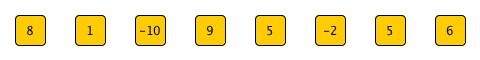The surpassers of an element are all elements whose indices are bigger and values are larger. For example, the element 1's surpassers are 9, 5, 5, and 6, so its number of surpassers is 4.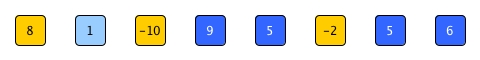And also we can see that 9 doesn't have any surpassers so its number of surpassers is 0.

So the problem of this pearl is:

Given an unsorted list of numbers, find the max number of surpassers, O(nlogn) is required.

In the answer for the above example is 5, for the element -10.

# An easy but not optimal solution

As usual, let's put a trivial solution on the table first (). It is straightforward:

1. For every element, we scan all elements behind it and maintain a ns as its number of surpassers.
2. If one element is larger, then we increase the ns.
3. After we finish on all elements, the max of all the nses is what we are looking for.

The diagram below demonstrates the process on 1.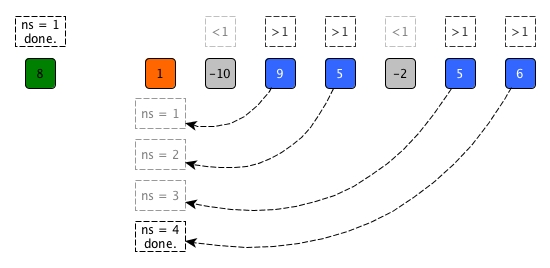let num_surpasser p l = List.fold_left (fun c x -> if x > p then c+1 else c) 0 l

let max_num_surpasser l =
let rec aux ms l =
match ms, l with
| _, [] -> ms
| None, p::tl -> aux (Some (p, num_surpasser p tl)) tl
| Some (_, c), p::tl ->
let ns = num_surpasser p tl in
if ns > c then aux (Some (p, ns)) tl
else aux ms tl
in
aux None l

(* note think the answer as an option will make the code seem more complicated, but it is not a bad practice as for empty list we won't have max number of surpassers *)


The solution should be easy enough to obtain but its time complexity is O(n^2) which is worse than the required O(nlogn).

# Introducing Divide and ConquerThe algorithm design technique divide and conquer was mentioned in Recursion Reloaded. I believe it is a good time to properly introduce it now as it provides a elegant approach towards a better solution for pearl 2.

## What is it, literally

Suppose we want to replace the dragon lady and become the king of the land below ().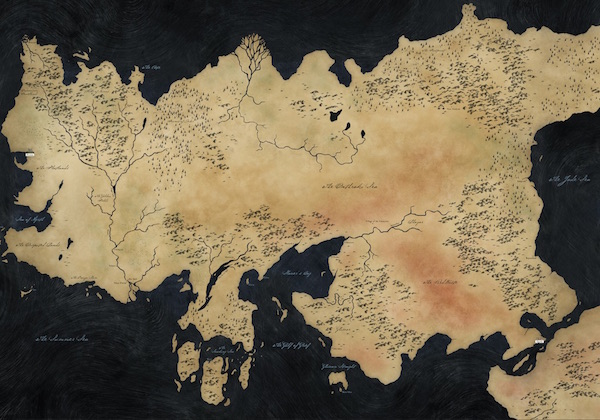We are very lucky that we have got a strong army and now the only question is how to overcome the realm.

One "good" plan is no plan. We believe in our troops so much that we can just let all of them enter the land and pwn everywhere.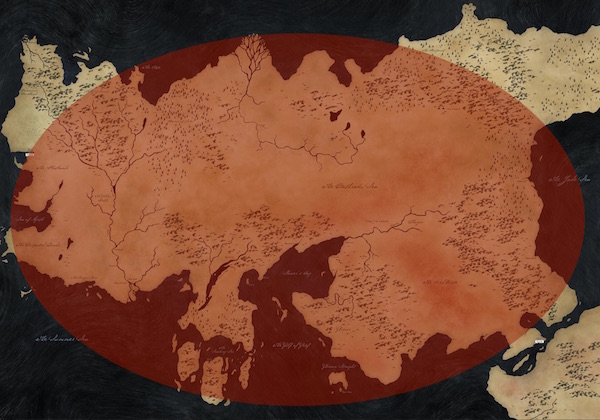Maybe our army is very good in terms of both quality and quantity and eventually this plan will lead us to win. However, is it really a good plan? Some soldiers may march to places that have already been overcome; Some soldiers may leave too soon for more wins after one winning and have to come back due to local rebel... the whole process won't be efficient and it cost too much gold on food for men and horses.

Fortunately, we have a better plan.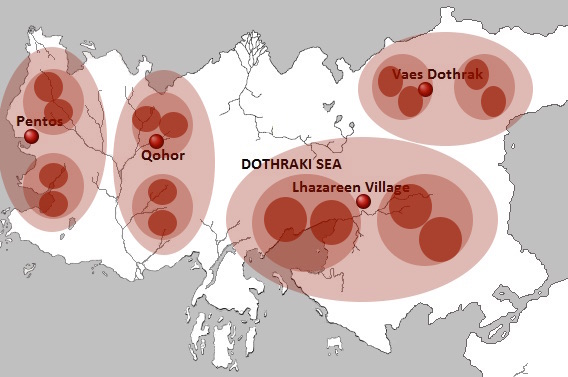We divide the land into smaller regions and further smaller ones inside until unnecessary. And for each small region, we put ideal amount of soldiers there for battles. After soldiers finish their assigned region, they don't need to move and just make sure the region stay with us. This is more oganised and more efficient in terms of both gold and time. After all, if we conquer all the tiny regions, who would say we were not the king?

## What is it in algorithm design, with accumulation

divide and conquer in algorithm design is not a universal solution provider, but a problem attacking strategy or paradigm. Moreover, although this classic term has been lasting for quite a long time, personally I would like to add one more action - accumulate - to make it appear more complete. Let's check the 3 actions one by one to see how we can apply the techque.

### Divide

Conceptually this action is simple and we know we need to divide a big problem into smaller ones. But how to is non-trivial and really context-sensitive. Generally we need to ask ourselves 2 questions first:

What are the sizes of the smaller sub-problems?

Normally we intend to halve the problem because it can lead us to have a O(logn) in our final time complexity.

But it is not a must. For example 3-way quicksort divides the problem set into 3 smallers ones. 3-way partition can let quicksort have O( $\log_3{n}$ ). However, do not forget the number of comparison also increases as we need to check equality during partition.

Moreover, sometimes we may have to just split the problem into a sub-problem with size 1 and another sub-problem with the rest, like what we did for the sum function. This kind of divide won't give us any performance boost and it turns out to be a normal recursion design.

Do we directly split the problem, or we somehow reshape the problem and then do the splitting?

In mergesort, we simply split the problem into 2; while in quicksort, we use partition to rearrange the list and then obtain the 2 sub-problems.

The point of this question is to bear in mind that we do not have shortcuts. We can have a very simple splitting, but later on we need to face probably more complicated accumulate. Like mergesort relies on merge. Or, we can do our important work during divide phase and have a straight accumulate (quicksort just needs to concatenate the solutions of the two sub-problems with the pivot in the middle).

### Conquer

This action implies two things:

1. Recursion. We divided the problem, then we need to conquer. How to conquer? We need to apply divide and conquer and accumulate again until we are not able to divide any more.
2. Edge cases. This means if we cannot divide further, then it is time to really give a solution. For example, let's say our target is a list. If we reach an empty list or an one-element list, then what shall we do? Normally, if this happens, we do not need to accumulate and just return the answer based on the edge cases.

I believe the conquer part in the original divide and conquer term also implies the accumulate. I seperate accumulate as explained next.

### Accumulate

After we conquer every little area of the land, we should now somehow combine all our accomplishments and really build a kingdom out of them. This is the step of accumulate.

A key way to figure out how to accumulate is to start from small. In mergesort, if each of the 2 sub-problems just has one element, then the according answer is a list having that element and we have finished the conquer step. Now we have two lists each of which has one element, how can we accumulate them to have a single sorted list? Simple, smaller element goes first into our resulting list. What if we have two sorted list each of which has two elements? The same, smaller element goes first again.

If we decide to divide the problem in a fairly simple way, then accumulate is normally non-trivial and also dominates the time complexity. Figuring out a cost-efficient approach of accumulate is very important.

### Summary

Again, divide and conquer and accumulate is just a framework for solving an algorithm problem. All the concrete solutions are problem context based and can spread into all 3 steps.

In addition, a fundamental hint to using this techqniue is that if we are given a problem, and we know the future solution is not anyhow related to the problem size, then we should try divide and conquer and accumulate

# Solve pearl 2

Although pearl 2 asks us to get the max number of surpassers, we can

1. Get the number of surpassers for every element (we anyway need to)
2. Then do a linear scan for the max one.

The second step is O(n). If we can achieve the first step in O(nlogn), the overall time complexity stays as O(nlogn).

So our current goal is to use divide and conquer and accumulate to get all numbers of surpassers.

# Divide the problem of pearl 2We have such as list and we want to get a new list that have the same elements and each element is associated with the number of its surpassers. Now we want to divide the original list (problem set).

Can we directly halve the list?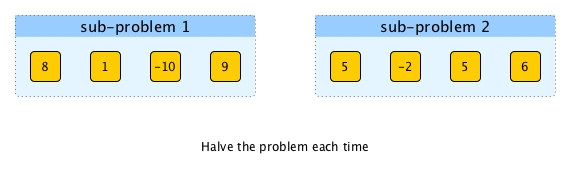As pearl 2 stated, an element only cares about all elements that are behind it. So if we split the list in the middle, we know the numbers of surpassers for all elements in sub-problem 2 do not need any special operations and the answers can directly be part of the future resulting list.

For the elements inside sub-problem 1, the answers are not fully accomplished yet as they will be affected by the elemnts in sub-problem 2. But hey, how we obtain full answers for sub-problem 1 with the help of the solutions of sub-problem 2 should be the job of accumulate, right? For now, I believe halving the problem is a good choice for divide as at least we already solve half of the problem directly.

# Conquer

We of course will use recursion. For sub-problems, we further halve them into smaller sub-problems until we are not able to, which means we reach the edge cases.

There are possibly two edge cases we need to conquer:

1. Empty list
2. A list with only one element.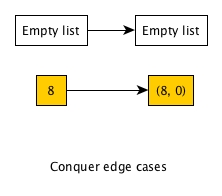For empty list, we just need to return an empty list as there is no element for us to count the number of surpassers. For an one-element list, we also return an one-element resulting list where the only element has 0 surpassers.

# Accumulate

Now we finished dividing and conquering like below and it is time to accumulate (take sub-problem 1 only for illustration).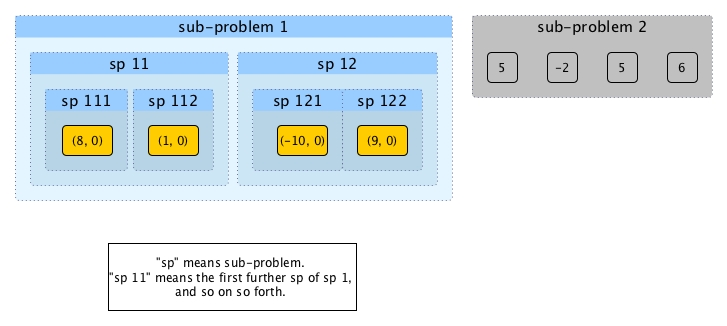It is easy to combine solutions of sp 111 and sp 112: just compare 8 from sp 111 with 1 from sp112, update 8's number of surpassers if necessary and we can leave sp 112 alone as we talked about during divide. The same way can be applied on sp 121 and sp 122. Then we get: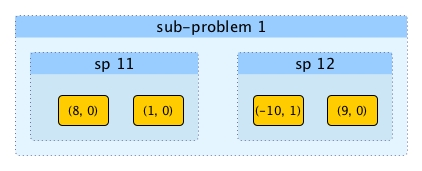Now both sp 11 and sp 12 have more than one element. In order to get the solution for sp 1, sp 12 can stay put. How about sp 11? An obvious approach is just let every element in sp 11 to compare every element in sp 12, and update their numbers of surpassers accordingly. This can be a candidate for accumulate action, however, it is O(n^2). We need to accumulate better.

We said in the very beginning of this post during our trivial solution that the original order of the list matters. However, is it still sensitive after we get the solution (for a sub-problem)?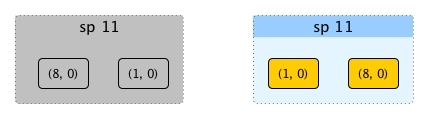As we can see once the answer of sp 11 is obtained, the order between 8 and 1 doesn't matter as they don't rely on each for their number of surpassers any more.

If we can obtain the solution in a sorted manner, it will help us a lot. For example, assume the resulting lists for sp 11 and sp 12 are sorted like this: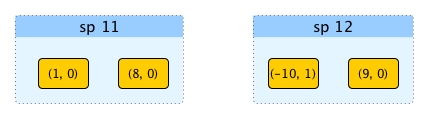Then we can avoid comparing every pair of elements by using merge like this: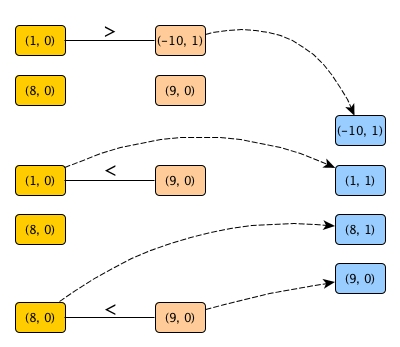We can see that 8 in the left hand side list doesn't have to compare to -10 any more. However, this example has not shown the full picture yet. If keep tracking the length of resulting list on the right hand side, we can save more comparisons. Let's assume both sp 1 and sp 2 have been solved as sorted list with lengths attached.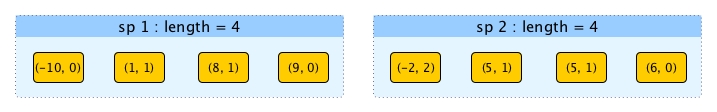We begin our merge.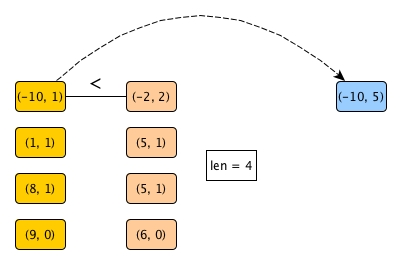Have you noticed the fascinating part? Because -10 < -2, without further going down along the resulting list on the right hand side, we can directly update the number of surpassers of -10 and get it out. Why? Because -2 is the smallest element on the right, and if it is bigger than -10, then the rest of the elements on the right must all be bigger than -10, right? Through only one comparison (instead of 4), we get the number of surpassers.

Thus, as long as the solutions of all sub-problems are sorted list with the length associated, we can accumulate like this:

1. Compare the heads hd1 and hd2, from two sorted resulting lists l1 and l2, respectively
2. If hd1 >= hd2, then hd2 gets out; go to 1 with updated length for l2
3. if hd1 < hd2, then hd1 gets out, and its ns gets updated by adding the length of l2 to the existing value; go to 1 with updated length for l1

The full process of accumulating sp 1 and sp 2 is illustrated as follows: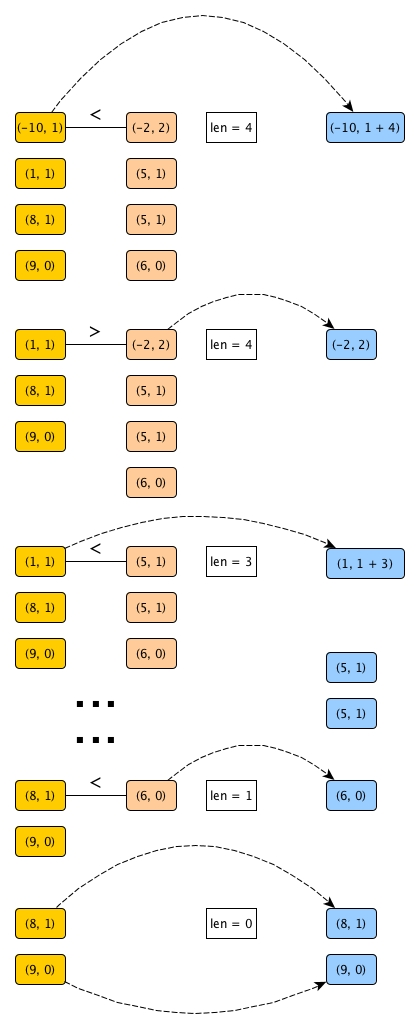Two things might need to be clarified:

1. Although we assumed the resulting lists of sub-problems to be sorted, they will naturally become sorted anyway because we are doing the smaller goes first merging.
2. We need to attach the lengths to each resulting list on the right and keep updating them because scanning the length of a list takes O(n).

Obviously this way of accumulation can give us O(n). Because at most we can divide O(logn) times, our divide and conquer and accumulate solution will be O(nlogn).

# Code

At first, we divide. Note that this version of divide is actually a kind of splitting from middle, as the original order of the elements before we get any solution is important.

(* we have a parameter n to indicate the length of l.
it will be passed by the caller and
in this way, we do not need to scan l for its length every time.

it will return left, right and the length of the right.
*)
let divide l n =
let m = n / 2 in
let rec aux left i = function
| [] -> List.rev left, [], 0
| right when i >= m -> List.rev left, right, n-i
| hd::tl -> aux (hd::left) (i+1) tl
in
aux [] 0 l


Now accumulate. We put it before writing conquer because conquer would call it thus it must be defined before conquer.

let accumulate l1 l2 len2 =
let rec aux acc len2 = function
| l, [] | [], l -> List.rev_append acc l
| (hd1,ns1)::tl1 as left, ((hd2,ns2)::tl2 as right) ->
if hd1 >= hd2 then aux ((hd2,ns2)::acc) (len2-1) (left, tl2)
else aux ((hd1,ns1+len2)::acc) len2 (tl1, right)
in
aux [] len2 (l1, l2)


conquer is the controller.

(* note the list parameter is a list of tuple, i.e., (x, ns) *)
let rec conquer n = function
| [] | _::[] as l -> l
| l ->
let left, right, right_len = divide l n in
let solution_sp1 = conquer (n-right_len) left in
let solution_sp2 = conquer right_len right in
accumulate solution_sp1 solution_sp2 right_len


So if we are given a list of numbers, we can now get all numbers of surpassers:

let numbers_of_surpassers l =
List.map (fun x -> x, 0) l |> conquer (List.length l)


Are we done? No! we should find the max number of surpassers out of them:

(* we should always consider the situation where no possible answer could be given by using **option**, although it is a bit troublesome *)
let max_num_surpassers = function
| [] -> None
| l ->
let nss = numbers_of_surpassers l in
Some (List.fold_left (fun max_ns (_, ns) -> max max_ns ns) 0 nss)


 Unless I can see an optimal solution instantly, I always intend to think of the most straightforward one even though it sometimes sounds stupid. I believe this is not a bad habit. Afterall, many good solutions come out from brute-force ones. As long as we anyway have a solution, we can work further based on it and see whether we can make it better.

 Yes, I believe the dragon lady will end the game and win the throne. It is the circle and fate.